# Test Statistics for Hypothesis Testing in Statistics with Functions in R Programming

1. Testing for the significance of the population mean (based on the sample or empirical observation)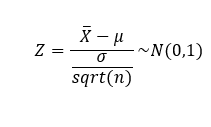test statistics for the significance of the population mean, when population variance known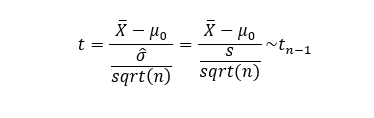test statistics for the significance of the population mean, when population variance unknown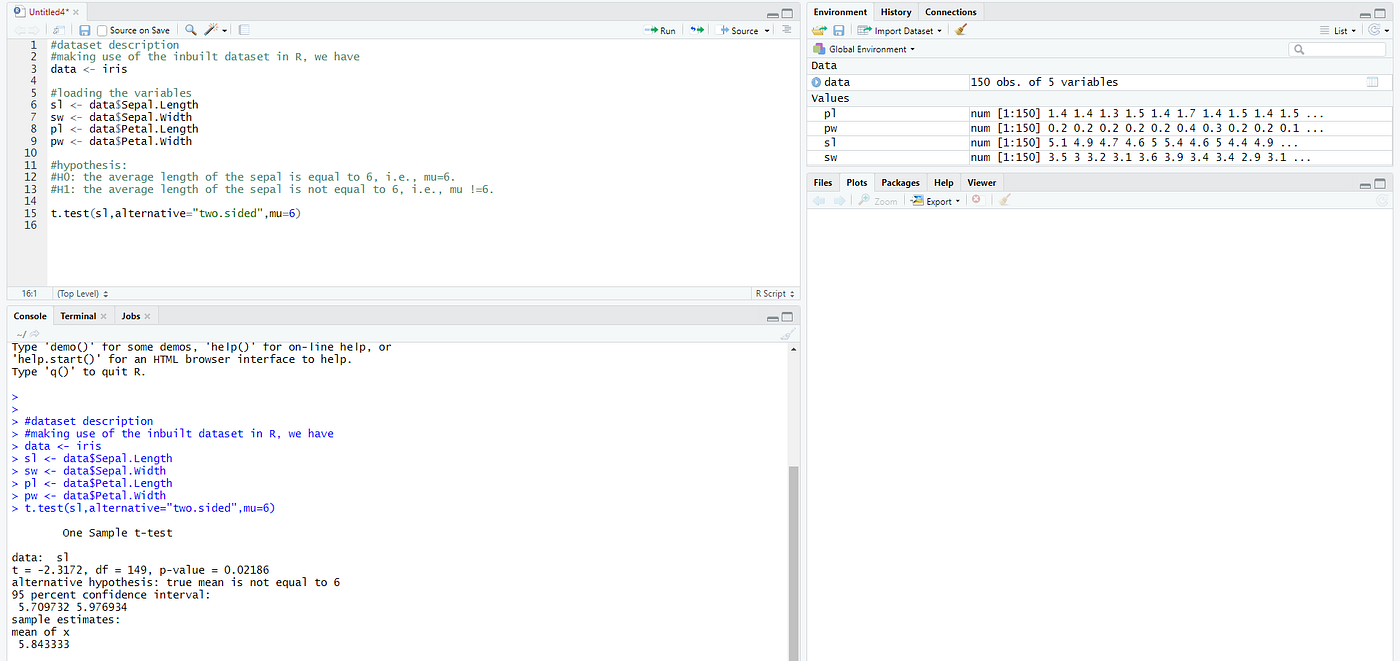the output screen for the r-code and the results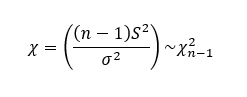test statistics for the significance of the population variance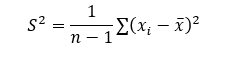formula to obtain the sample value of S²

--

--